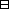## Group Theory and Linear Algebra

Last updated: 24 September 2014

## Lecture 26: Centres and $p\text{-groups}$

Let $p$ be a prime in ${ℤ}_{>0}\text{.}$

A $p\text{-group}$ is a group $G$ such that there exists $a\in {ℤ}_{>0}$ with $\text{Card}\left(G\right)={p}^{a}\text{.}$

Let $G$ be a $p\text{-group,}$ $\text{Card}\left(G\right)={p}^{a}\text{.}$

 (a) $G$ contains an element of order $p\text{.}$ (b) $𝒵\left(G\right)\ne \left\{1\right\}\text{.}$Proof.

 (a) To show: There exists $y\in G$ with $\text{order}\left(y\right)=1\text{.}$ Let $x\in G$ with $x\ne 1\text{.}$ Then $\text{order}\left(x\right)$ divides $\text{Card}\left(G\right)$ and $\text{order}\left(x\right)\ne 1\text{.}$ So $\text{order}\left(x\right)={p}^{b}$ with $0 Let $y={x}^{{p}^{b-1}}\text{.}$ Then $y\ne 1$ and $yp= (xpb-1)p =xpb=1.$ So $\text{order}\left(y\right)=p\text{.}$ (b) To show: $𝒵\left(G\right)\ne \left\{1\right\}\text{.}$ We know that $𝒵\left(G\right)$ is the union of the conjugacy classes of size $1\text{.}$ We know that, if ${𝒞}_{s}$ is a conjugacy class in $G$ then $Card(𝒞s)= Card(GStab(s)) divides Card(G)=pa.$ So, either $\text{Card}\left({𝒞}_{s}\right)=1$ or $\text{Card}\left({𝒞}_{s}\right)$ is divisible by $p\text{.}$ Then $pa=Card(G)= 1+1+⋯+1 ⏟number of conj.classes of size 1 + ∑conj. classes 𝒞swith Card(𝒞s)>1 Card(𝒞s).$ So (number of conjugacy classes of size 1) is divisible by $p\text{.}$ So $\text{Card}\left(𝒵\left(G\right)\right)$ is divisible by $p\text{.}$ So $𝒵\left(G\right)\ne \left\{1\right\}\text{.}$

$\square$

Let $G$ be a group with $\text{Card}\left(G\right)={p}^{2}\text{.}$ Then $G$ is abelian.Proof. To show: $𝒵=𝒵\left(G\right)$ is all of $G\text{.}$ We know, from (b) of the last Proposition, that $𝒵\ne \left\{1\right\}\text{.}$ We know, $\text{Card}\left(𝒵\right)$ divides $\text{Card}\left(G\right)={p}^{2}\text{.}$ So $\text{Card}\left(𝒵\right)=p$ or $\text{Card}\left(𝒵\right)={p}^{2}\text{.}$ Case 1: $\text{Card}\left(𝒵\right)=p\text{.}$ Let $x\in G$ with $x\notin 𝒵\text{.}$ Then $x𝒵$ generates $G𝒵= { 𝒵,x𝒵,x2𝒵,…, xp-1𝒵 }$ $\text{(}𝒵$ is a normal subgroup of $G$ and $G}{𝒵}\simeq ℤ}{pℤ}\text{).}$ Let $g\in G\text{.}$ Then there exists $0\le k and $z\in 𝒵$ with $g=xkz.$ So $gx = xkzx= xkxz,since z∈𝒵 = xk+1z= x(xkz) =xg.$ So $x\in 𝒵\text{.}$ This is a contradiction to $x\notin 𝒵\text{.}$ So $\text{Card}\left(𝒵\right)\ne p\text{.}$ Case 2: $\text{Card}\left(𝒵\right)={p}^{2}\text{.}$ Since $\text{Card}\left(G\right)={p}^{2},$ then $𝒵=G\text{.}$ So $G$ is abelian. $\square$

### About conjugacy classes, normal subgroups and centres

(1) Let $N$ be a normal subgroup of $G\text{.}$ Then $N$ is a union of conjugacy classes of $G\text{.}$Proof. To show: If $n\in N$ then ${𝒞}_{n}\le N\text{.}$ To show: If $n\in N$ and $g\in G$ then $gn{g}^{-1}\in N\text{.}$ This is true since $N$ is normal. $\square$

(2) Let $G$ be a group and $𝒵=𝒵\left(G\right)\text{.}$ Then $𝒵$ is a normal subgroup of $G$ and if $z\in 𝒵$ then ${𝒞}_{z}=\left\{z\right\}\text{.}$Proof. To show: If $z\in 𝒵$ then ${𝒞}_{z}=\left\{z\right\}\text{.}$ Assume $z\in 𝒵\text{.}$ To show: ${𝒞}_{z}=\left\{z\right\}\text{.}$ $𝒞z= {gzg-1 | g∈G}= {gg-1z | g∈G}= {z}.$ $\square$

If ${𝒞}_{z}=\left\{z\right\}$ then $z\in 𝒵\text{.}$Proof. Assume $z\in G$ and ${𝒞}_{z}=\left\{z\right\}\text{.}$ To show: $z\in 𝒵\text{.}$ To show: If $g\in G$ then $gz=zg\text{.}$ Assume $g\in G\text{.}$ Then $gz=\left(gz{g}^{-1}\right)g=zg,$ since $gz{g}^{-1}\in {𝒞}_{z}=\left\{z\right\}\text{.}$ $\square$

## Notes and References

These are a typed copy of Lecture 26 from a series of handwritten lecture notes for the class Group Theory and Linear Algebra given on October 5, 2011.